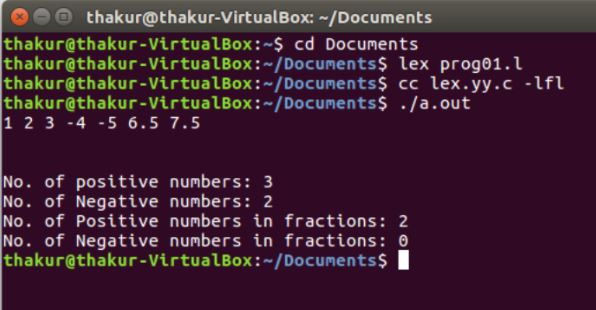# Lex program to Count the Positive numbers, Negative numbers and Fractions

• Last Updated : 01 May, 2019

Problem : Write a Lex program to count the positive numbers, negative numbers and fractions

Explanation :
FLEX (Fast Lexical Analyzer Generator) is a tool/computer program for generating lexical analyzers (scanners or lexers) written by Vern Paxson in C around 1987. Lex reads an input stream specifying the lexical analyzer and outputs source code implementing the lexer in the C programming language. The function yylex() is the main flex function which runs the Rule Section.

Prerequisite: Flex (Fast lexical Analyzer Generator)

Examples:

```Input: 2 -8 -2.5 8.5
Output: No. of positive numbers: 1
No. of Negative numbers: 1
No. of Positive numbers in fractions: 1
No. of Negative numbers in fractions: 1

Input: 1 2 3 -4 -5 6.5 7.5
Output: No. of positive numbers: 3
No. of Negative numbers: 2
No. of Positive numbers in fractions: 2
No. of Negative numbers in fractions: 0 ```

Implementation:

 `/* Lex program to Count the Positive numbers, ``      ``- Negative numbers and Fractions  */`` ` `%{``     ``/* Definition section */``    ``int` `postiveno=0;``    ``int` `negtiveno=0;``    ``int` `positivefractions=0;``    ``int` `negativefractions=0;``%}`` ` `/* Rule Section */``DIGIT [0-9]``%%`` ` `\+?{DIGIT}+             postiveno++;``-{DIGIT}+               negtiveno++;`` ` `\+?{DIGIT}*\.{DIGIT}+   positivefractions++;``-{DIGIT}*\.{DIGIT}+     negativefractions++;``. ;   ``%%`` ` `// driver code``int` `main()``{``    ``yylex();``    ``printf``(``"\nNo. of positive numbers: %d"``, postiveno);``    ``printf``(``"\nNo. of Negative numbers: %d"``, negtiveno);``    ``printf``(``"\nNo. of Positive numbers in fractions: %d"``, positivefractions);``    ``printf``(``"\nNo. of Negative numbers in fractions: %d\n"``, negativefractions);``    ``return` `0;``}`

Output:My Personal Notes arrow_drop_up